CLAT  >  Test: Time And Distance- 2

# Test: Time And Distance- 2 - CLAT

Test Description

## 20 Questions MCQ Test Quantitative Techniques for CLAT - Test: Time And Distance- 2

Test: Time And Distance- 2 for CLAT 2023 is part of Quantitative Techniques for CLAT preparation. The Test: Time And Distance- 2 questions and answers have been prepared according to the CLAT exam syllabus.The Test: Time And Distance- 2 MCQs are made for CLAT 2023 Exam. Find important definitions, questions, notes, meanings, examples, exercises, MCQs and online tests for Test: Time And Distance- 2 below.
Solutions of Test: Time And Distance- 2 questions in English are available as part of our Quantitative Techniques for CLAT for CLAT & Test: Time And Distance- 2 solutions in Hindi for Quantitative Techniques for CLAT course. Download more important topics, notes, lectures and mock test series for CLAT Exam by signing up for free. Attempt Test: Time And Distance- 2 | 20 questions in 30 minutes | Mock test for CLAT preparation | Free important questions MCQ to study Quantitative Techniques for CLAT for CLAT Exam | Download free PDF with solutions
 1 Crore+ students have signed up on EduRev. Have you?
Test: Time And Distance- 2 - Question 1

### A car covers first half of its journey at the rate of 15km/h and second half at the rate of 12km/h and total time taken to complete the journey is 9 hours. Find the length of journey.

Test: Time And Distance- 2 - Question 2

### A bus complete a certain journey in 19 hours. It covers first half of the distance at a rate of 15 km/h and second half at the rate of 20 km/h. The length of journey is …………

Test: Time And Distance- 2 - Question 3

### A student walks from his house at 2 km/h and reaches school 5 minutes late and if his speed had been 3 km/h he would have reached the school 5 minutes earlier. How far is his school from house?

Detailed Solution for Test: Time And Distance- 2 - Question 3

Speed = Distance/Time
Let ideal time taken to reach school is t, distance to school = distance(d)
With a speed of 4km/hr or 4/60 km/min he reaches 5 mins late i.e.
4/60= d/(t+5)
60d = 4t + 20 ----- (1)
Similarly
5/60 = d/(t-10)
60d = 5t - 50 ----- (2)
From eq 1 and 2
4t +20 = 5t - 50
t = 70 mins
60d = 4t + 20 = 280 + 20 = 300
distance(d) = 5 kms

Test: Time And Distance- 2 - Question 4

A person walks from his house at the rate of 6 km/h and reaches office 12 minutes late and if his speed had been 8 km/h he would have reached the office 8 minutes earlier. How far is his office from house?

Test: Time And Distance- 2 - Question 5

By walking 3/2 of his usual speed a person reach his office 10 minutes earlier. Find his usual time.

Test: Time And Distance- 2 - Question 6

Byof  2/5 his usual speed a student reach his school 30 minutes late. Find his usual time?

Test: Time And Distance- 2 - Question 7

A train whose length is 300m long is moving at the rate of 108 km/h. How long will it take to pass a pole?

Test: Time And Distance- 2 - Question 8

How long does a train 200 m long moving at the rate of 72 km/h take to pass a tunnel 40 m long?

Test: Time And Distance- 2 - Question 9

How long does a train 600 m long moving at the rate of 144 km/h take to pass a bridge 200 m long?

Test: Time And Distance- 2 - Question 10

Two trains of 400 m and 350 m long are running on parallel tracks at the rate of 180 km/h and 120 km/h respectively. How long will they take to cross each other if they are running in opposite direction?

Test: Time And Distance- 2 - Question 11

Two trains 500 m and 200 m long are running on parallel tracks at the rate of 160 km/h and 200 km/h. How long the second train take to cross 1st train if they are running in same direction.

Test: Time And Distance- 2 - Question 12

A train crosses a platform of length 350 m in 12 sec and a man standing on platform in 2 sec. Find the speed of train.

Detailed Solution for Test: Time And Distance- 2 - Question 12

The correct answer is B as
Let the length of train be x m.
Since the train crosses the man in 2 sec
Speed of train = x/2 m/s
Also it crosses the platform of length 350 m in 12 sec
So speed of train = (350+x)/12
Equating both the speeds of train ,we get
x/2 = (350+x)/12
x = (350+x)/6
6x = 350+x
5x = 350
x = 70
Thus length of train = 70 m
Now, speed of train = 70/2 = 35 m/s
= 35/1000*3600 = 35/5*18
=126 km/hr.

Test: Time And Distance- 2 - Question 13

A man moves downstream at a rate of 14 km/h and upstream at a rate of 6 km/h. Find his speed in still water.

Test: Time And Distance- 2 - Question 14

A man moves downstream at a rate of 14 km/h and upstream at a rate of 6 km/h. Find his speed in still water.

Test: Time And Distance- 2 - Question 15

A boat moves down the stream at the rate of 20 km/h and it takes 12 minutes for him to move one km against it. Find the speed of stream.

Test: Time And Distance- 2 - Question 16

A man can swim 4 km/h in still water. If the velocity of stream is 2 km/h, the time taken by him to swim to a place 12 km upstream and back is:

Test: Time And Distance- 2 - Question 17

A man can row three quarter of a km against the stream in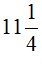min. and return in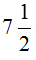min. The speed of man in still water is.

Detailed Solution for Test: Time And Distance- 2 - Question 17

Downstream = (3/4) (15/2)km/min = 1/10 =6km/hr
upstream = (3/4) ( 45/4) km/min =1/15 =4km/he
Stillwater = 1/2(6+4)= 5 km/hr

Test: Time And Distance- 2 - Question 18

A man takes twice as long to row a distance against the stream as to row the same distance in favour of the stream. The ratio of the speed of the boat (in still water) and the stream is:

Detailed Solution for Test: Time And Distance- 2 - Question 18

Let speed upstream = x
Then, speed downstream = 2x

Speed in still water =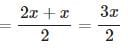Speed of the stream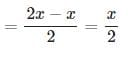Speed in still water : Speed of the stream  =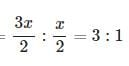Test: Time And Distance- 2 - Question 19

The speed of a boat upstream is 10 km/h. The speed of stream is 5 km/h. The speed of boatdown stream is.

Test: Time And Distance- 2 - Question 20

A thief steals a car at 9 am and drive at the rate of 20 km/h. The theft is discovered and police starts chasing him at 9.30 am. in a jeep at the speed of 40 km/h. The thief will be caught at ………

Detailed Solution for Test: Time And Distance- 2 - Question 20

The  correct answer is A as
Relative speed = 40-20 (same distance)
Distance = 10 KM
Time = distance/time = 10/20 = 1/2 hours = 30 minutes
9.30 + 30 = 10 AM

## Quantitative Techniques for CLAT

55 videos|35 docs|98 tests
Information about Test: Time And Distance- 2 Page
In this test you can find the Exam questions for Test: Time And Distance- 2 solved & explained in the simplest way possible. Besides giving Questions and answers for Test: Time And Distance- 2, EduRev gives you an ample number of Online tests for practice

## Quantitative Techniques for CLAT

55 videos|35 docs|98 tests(Scan QR code)